Try it now!

USD
10

# Company Valuation Report: CapitalMall Trust – Part 3

2054

## Part 2: Valuation of the Company

### 2.1 Price/Earnings Ratios

P/E Ratio is a measurement of market price of the stock against the expected earning that will derive from it. This P/E Ratio is also commonly referred to as the multiplier for expected earnings. Generally, the P/E ratio signifies the demand of current investors in a company share. For example, when we expect the P/E ratio of stock A to be 20, it would mean that for \$1 of current earnings per share, investors were willingly to pay \$20. A higher P/E ratio will allow a company to grow its earnings over time due to its sustainable advantage.

The P/E Ratio is not only an indicator of demand but also an indicator of company’s ability and market performance. Inversely, a lower P/E Ratio will mean that the company takes a shorter period to return investors their original invested capital. For example, if the company has a P/E Ratio of 10 & the original investment amount is \$100, this will mean that the company will take 10 years to add up their earnings to return investor his original investment, assuming everything else constant.

(P/E) ratio = Market price of the stock / earnings per share.

Do you require a dissertation proposal help? Our company gives you an opportunity to use excellent creative writing dissertation proposal service, which is affordable for absolutely everyone. Just contact our writers online!

There are a number of rationales for using the price to earnings ratio in valuation:

• Earnings power, as measured by earning per share(EPS), is the primary determinant of investment value
• The PE ratio is popular in the investment community

Empirical research have also shown that PE differences are significantly related to the long run average stock return.

 PE Ratio Calculation 2009 2010 2011 2012 Profit after tax -65 270 384 536 No. of Shares outstanding 2921 3182 3206 3567 EPS -0.02 0.084 0.12 0.15 Market Value of Share (yearly Average) 1.27 1.58 1.64 1.79 PE Ratio (Price/EPS) -63.5 18.8 13.67 11.92

Analyzing PE Ratio Results:

Our PE Calculations above indicate that over the years the PE ratio of the company was -63.5, 18.8, 13.67 and 11.92, respectively. In other words, since 2010, the PE ratio is declining which indicates that the company is capable to return the invested capital in short term. Hence, the PE valuation is favorable to nominate the company under ‘Buy’ Category.

### 2.2 Price to Book Value Ratio

Price-Book Value Ratio (P/BV) is also known as the price/equity ratio, widely used to compare companies that have consistent accounting practice (Reilly and Brown, 2009). This ratio varies with industries. This ratio compares the company’s current market value to its book value. The book value is the total of capital invested by the shareholder and the amount of retained earnings, reserves and minority interest.

A higher P/B ratio implies that the stock may be overvalued. This meant that investors would expect that management would create more values from a given set of assets holding all else equal. A lower P/B ratio, on the other hand, could mean that the stock may be undervalued. Alternatively, it could mean that something is fundamentally wrong with the company. A low P/B value indicates a value stock while a high P/B value indicates a growth stock. Investors seek out for value stocks as they believe it is underpriced, this will lead to an increase in market demand for the stock. Once the market realizes the real value of the stock, the price of the stock will increase and investors will receive a reasonable amount of profit. This ratio however, does not provide any information on the ability of the firm to generate profits for the shareholders. Hence, before proceeding with the Price/Book Value Calculation, it will be advantageous for us to discuss pros and cons of this ratio multiple:

Pros:

• Book Value is a cumulative amount that is usually positive, even when the firm reports a loss and EPS is negative. Thu, P/B ratio can be used where P/E turns ineffective.
• Book Value is more stable than EPS, so it may be more useful than the P/E when EPS is particularly high, low or volatile.
• Book Value is an appropriate measure of net asset value for firms that primarily hold liquid assets. Example includes finance, investments, insurance and banking firms.
• Empirical Research has also shown that P?B ratios are helpful in explaining the differences in long run average stock returns
• P/B ratios are also helpful in valuation of the companies that are expected to go out of business.

Cons:

• P/B Ratios do not reflect the value of the intangible economic assets, such as human capital.
• P/B can be misleading when there are significant differences in the asset size of the firms under consideration because in some of the cases, the firm’s business model dictates the size of its asset base. A firm that outsources its production will have fewer assets, lower book value and a higher P/B ratio than an otherwise similar firm in the same industry that doesn’t outsource.
• Inflation and technological changes can cause the book and market values of assets to differ significantly, so book value is not an accurate measure of the value of shareholders investments. This makes it more difficult to compare P/B across firms

P/B Ratio is calculated using a simple formula, which is given below: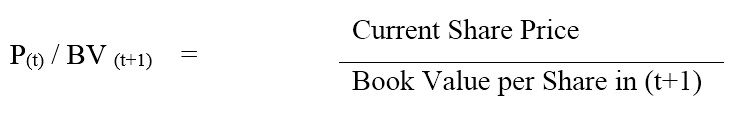Here Book Value of the company refers to Book Value of Equity

So, Book Value per Share= Book Value per Equity/ Number of shares outstanding

Evaluation of validity of this figure has been compared with its historical values:

 P/B Calculation 2009 2010 2011 2012 Book Equity 4970 4939 5246 5703 Outstanding Shares 2921 3182 3206 3567 Market price(yearly average) 1.27 1.58 1.64 1.79 Book Value per share 1.7 1.552 1.636 1.59 P/B Ratio 0.74 1.018 1.002 1.125

Analyzing the P/B Ratio:

Our above calculation indicates that the Price/ Book Value ratio of CapitaMall Truet has been consistently increasing over the years. This may be the result of positive outlook in the Real Estate Industry after the end of global economic crisis. The increase in price to book value ratio of the company from 0.74 during 2009 to 1.125 during 2012 can be attributed to the growing confidence of the investors in the company’s operations.

Hence, once again, using this ratio multiple, we can provide a ‘Buy’ rating for the company’s stock.### 2.3 Beta Valuation

Beta is commonly used for measuring of risk of an asset relative to the market or benchmark factor. It is used to establish a portfolio’s volatility in relative to the market. Hence, beta values are used to gauge the sensitivity of the rate of return for a given stock to that of the market’s rate of return. It is commonly used to calculate systematic risks. Systematic risk refers to the market risk, which cannot be eliminated through diversification.

Beta is calculated using the regression analysis. Beta value of more than 1 means the security is more volatile in the market. Therefore, there will be a larger asset price movement in relation to a trivial change in the market returns. For example, a beta value of 1.5% means that there will be a 1.5% change in the rate of return of an asset for every 1% change in the market return. Alternatively, a beta value of less than 1 means there is more security stability and less volatile in the market. A trivial change in the market returns will cause a less responsive change in the price of an asset.

Beta can be calculated using either the given formula: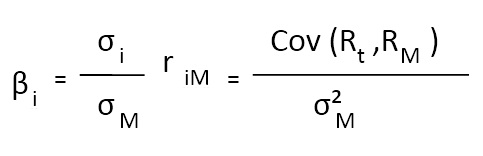Where:

Ri = excess return of the asset (Ri = ri – rf)

Rm = excess return of the market portfolio (RM = rM - rf)

αi = expected excess return of the asset when Rm=0

ei = company-specific error term representing unexpected returns;

E (ei)=0

Alternatively, the second approach to the valuation of beta is by using regression analysis using annualized returns of CMT’s stock and SIT Index. Important to note, the regression approach is preferred to the formula approach as it measures the statistical reliability of the estimated results. We will use STI as a proxy for the risky market portfolio for our calculation. STI is the market value-weighted stock market index, which consists of stocks from 30 listed companies on the Singapore Exchange.

The detailed regression results are disclosed below:

Regression Results: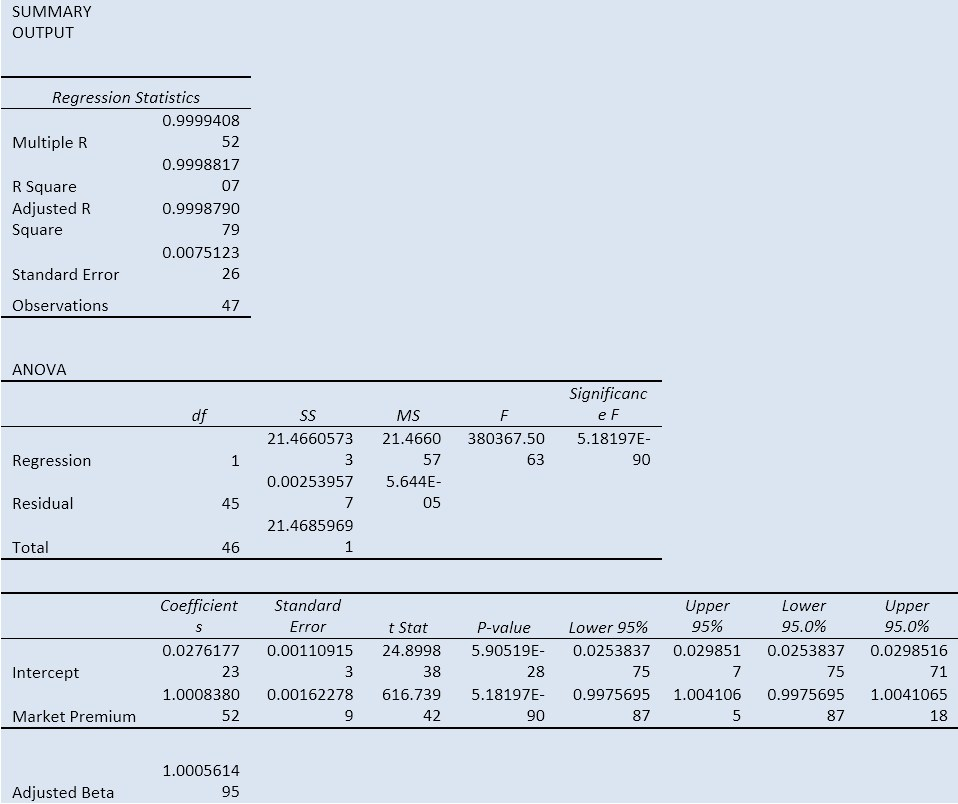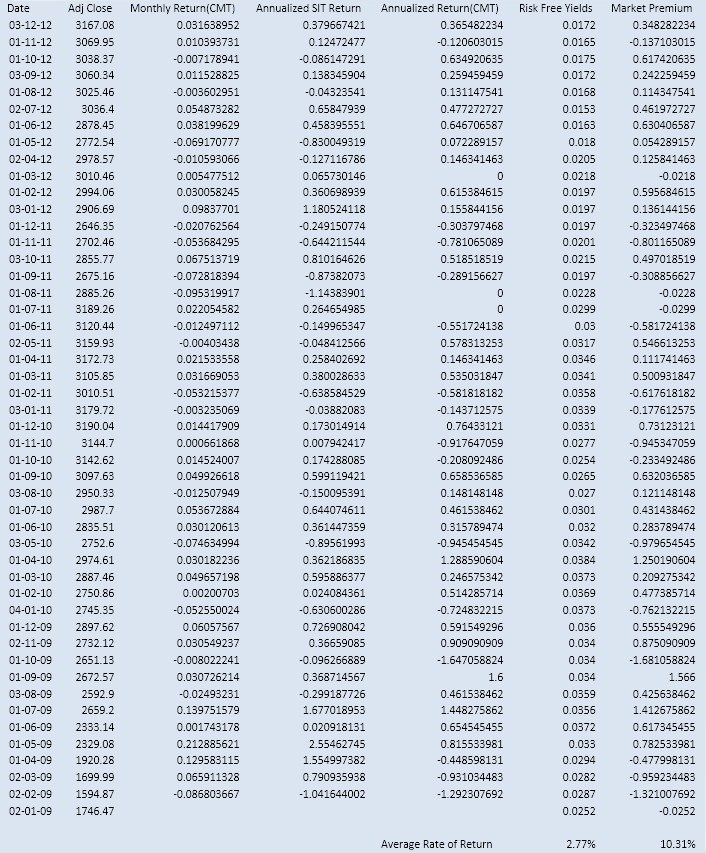** In order to adjust the beta, we have used the following equation:

β adj = (0.67)βraw + 0.33

So, adjusted beta = 0.67(1.003) + 0.33

= 1.00201

Risk-Free Rate

For the purpose of calculation of Expected Return using CAPM Model, we will be using the average annualized yield of Singapore 10 Year Treasury bonds. Thus, the average rate of 2.77% will be used as the proxy for the risk free rate of return.

Market risk premium is a key component of CAPM and is defined as the difference between the expected future return on the overall stock market and the current risk-free rate. The premium represents the compensation risk-adverse investors requires for investing in riskier market portfolios.

We assume that overall domestic macroeconomic performance will be the key driver for returns on market portfolio in determining the expected market return. This means that the key driver behind market returns will be changes in gross domestic product (GDP).

For the purpose of this report, we will be using the average market premium rate by subtracting the annualized return of SIT from the risk free rate of treasury yields. The average market premium rate will be 10.31%

Assumptions:

• Market portfolio accurately reflects the performance in the market.
• Market Risk Premium can be derived by using the expect market return to subtract the risk free.

Capital Asset Pricing Model (CAPM)

To calculate the expected return from CMT’ stock using CAPM, we will use the standard CAPM formula: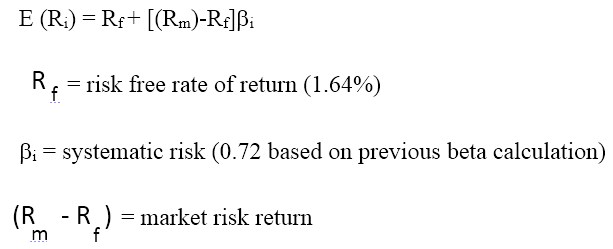E(R) = 2.77 + 1.0056(10.31)

E(R) = 13.13%

Analyzing the beta valuation:

Our regression was based on a total observation of 48 observations from January 2009 to December 2012. Our regression analysis produced a raw beta of 1.008. Thus, we can we can infer that the beta is almost close to the market. In other words, the volatility of the share price of Capitamall Trust is almost as similar as of STI Index. Thus, a 1% change in STI Index will cause similar change in CMT’s price. Thus, since the stock is following the market index volatility, it will be most appropriate to hold the stock.

ii) Dividend Discount Model:

Valuation of the companies by discounting the dividends is one of the most popular models in the equity valuation world. The primary advantage of using the dividends as the definition of cash flows is that it is theoretically justified. The shareholder’s investment today is worth the present value of the future cash flow that he expects to receive, and ultimately he will be repaid for his investment in the form of dividends. Even if the investor sells the stock at any time prior to the liquidation of the company, before all the dividends are paid, he will receive from the buyer of the shares the present value of the expected future dividends.

An additional advantage of dividends as a measure of the cash flows is that the dividends are less volatile than other measures and therefore the value estimates derived from dividend discount models are less volatile and reflect the long term earning potential of the company.

For the purpose of valuation of the company using dividend discounting methods, we shall be using Gordon Growth model. This model assumes that the dividends increase at a constant rate indefinitely. The simplifying factor of the constant growth assumption is that the rate of growth can be expressed per period in the same way that the required return is expressed allowing the expression to be condensed into a simple formula:

V= D0 (1+g)/ (k-g)

Here:

V= Fundamental Value

D0= Present Dividend

R= Required rate on equity

G= Dividend Growth Rate

The model assumes that:

• The firm expects to pay dividends in one year
• Dividend grow indefinitely at a constant rate, g
• The growth rate will be less than the required rate on equity

Dividend Discount Model (DDM) for CapitaMall Trust:

DDM  FY13 FY14F FY15F FY16F Terminal Value

DPU (SG cts): 10.31 10.74 10.93 11.14 230.66

Fair Value: 2.10

Current Price: 1.955

Price Upside (%): 7%

Distribution Yield (%): 5%

Total Return (%): 13%

Important Assumptions:

Risk-free rate: 3%

Beta: 0.69

Cost of Equity: 6.8%

Terminal Growth: 2%

Thus, using the DDM Valuation, the Fair value of the company is S\$2.10

DDM Valuation Analysis:

Under some assumptions, the intrinsic valuation of the company is S\$2.10, which means that the stock is undervalued and will be a good investment to buy for the investors.

Whether you cannot start, finish, or edit your essay, we are always here to help you! Ask for persuasive essay help at Pro-Papers and get ready to make your teacher proud of you.

## Works Cited

CapitalMall Trust. (2013). Annual Report 2013. Singapore: CapitalMall Trust.

CapitalMall Trust. (2014, April 4). Retrieved from Capitalmall.com: http://www.capitamall.com/home/home.html

Gabriel, A. (2014, April 4). Bearish outlook for Reits in 2014, says analysts. Retrieved from Asia One Business: http://business.asiaone.com/news/bearish-outlook-reits-2014-says-analysts/page/0/1

Jacintha Poh, P. L. (2014, April 4). Moody's: Outlook for S-REIT sector is stable in 2014. Retrieved from Moodys.com: https://www.moodys.com/research/Moodys-Outlook-for-S-REIT-sector-is-stable-in-2014--PR_288265

Zacks.com. (2014, April 4). REIT Industry Review & Stock Picks - Feb 2014 - Industry Outlook. Retrieved from Nasdaq.com: http://www.nasdaq.com/article/reit-industry-review-stock-picks-feb-2014-industry-outlook-cm329901Leave a Reply